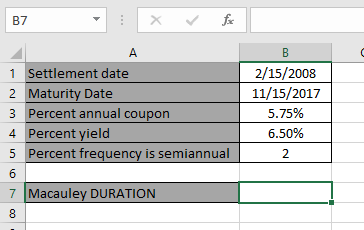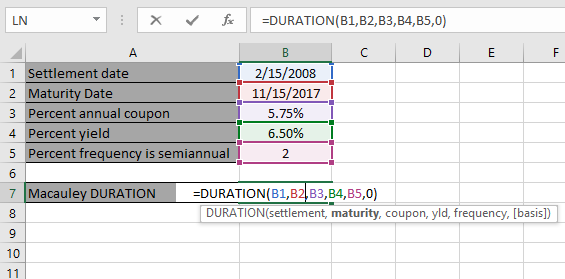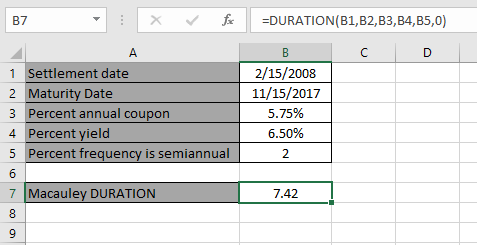# How to use the DURATION Function in Excel

The DURATION function is a built-in financial function which returns the Macauley duration per \$100 face value of a security that pays periodic interest.
Syntax:

=DURATION(settlement, maturity, rate, yld, redemption, frequency, [basis])

Settlement : Settlement date of the security
Maturity : Maturity date of the security. The maturity date is the date when the security expires.
Rate : The security's annual coupon rate
Yld : The security's annual yield
Frequency : Coupon payments per year (annual = 1, semiannual = 2; quarterly = 4.
basis : [optional] Day count basis.

Let’s understand this function using it in an example.Here we have values to calculate the Macauley DURATION per \$100 face value of a security that pays periodic interest.

Use the formula:

=DURATION(B1, B2, B3, B4, B5, 0)

B1 : Settlement date of the security
B2 : Maturity date of the security. The maturity date is the date when the security expires.
B3 : The security's annual coupon rate
B4 : The security's annual yield
B5 : Coupon payments per year (semiannual = 2).
0 : [optional] Day count basis.Note: Dates in the formula must be entered using cell reference or DATE function. Excel date format creates #VALUE! error while computing results. Use the cell reference or DATE function in excel while taking date as input to the function

Now Press Enter to get the DURATIONThe DURATION is 7.42

As you can see the formula returns the DURATION per \$100 face value of a security that pays periodic interest.

Hope you understood how to use DURATION function and referring cell in Excel. Explore more articles on Excel function here. Please feel free to state your query or feedback for the above article.

Related Articles:

How to use the DURATION Function in Excel

How to use the Excel IPMT Function

How to use Compound Interest Function in Excel

Popular Articles:

50 Excel Shortcuts to Increase Your Productivity

How to use the VLOOKUP Function in Excel

How to use the COUNTIF in Excel 2016

How to use the SUMIF Function in Excel

Terms and Conditions of use

The applications/code on this site are distributed as is and without warranties or liability. In no event shall the owner of the copyrights, or the authors of the applications/code be liable for any loss of profit, any problems or any damage resulting from the use or evaluation of the applications/code.